Problem

# A crane consists of a quarter-circular bar that lies in a plane parallel to the xz plane...

A crane consists of a quarter-circular bar that lies in a plane parallel to the xz plane with a low-friction collar at point B that may slide on the bar. The forces supported by cables AB and BC are 30 lb and 1500 lb, respectively, and the coordinates of point A are given in the figure. For the value of angle α given below, determine expressions for the forces the two cables apply to the collar at point B.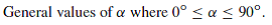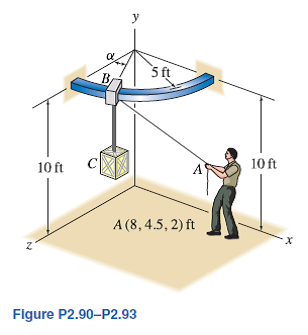#### Step-by-Step Solution

Solution 1

Draw the forces supported by cables AB and BC as follow.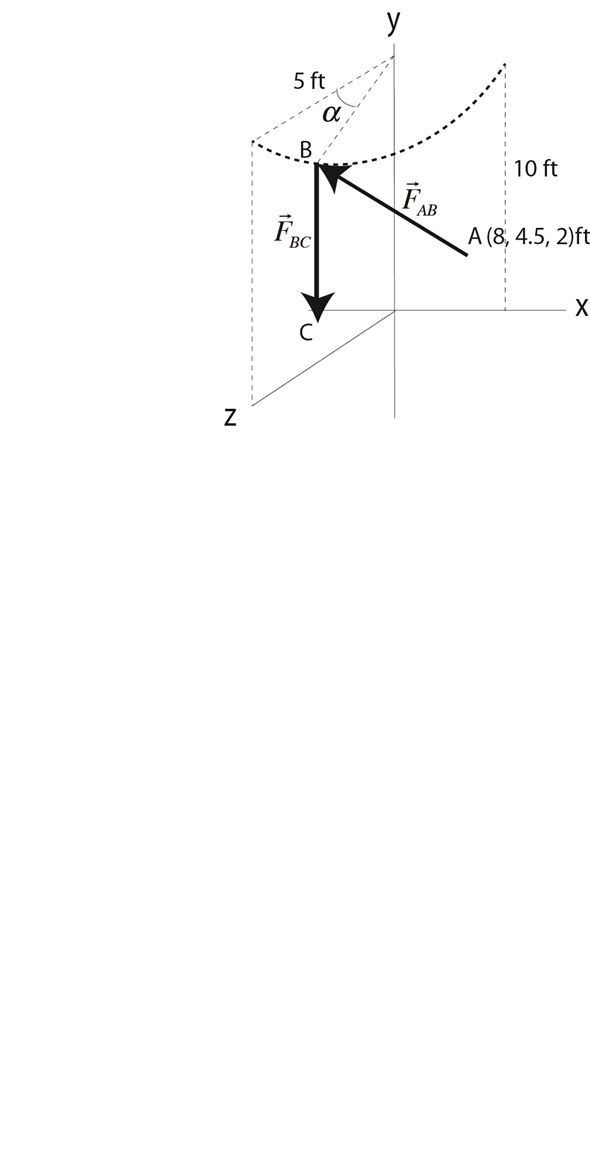For $$\boldsymbol{\alpha}=\mathbf{0}^{\circ}$$ :

Determine the position vector between $$A$$ and $$B$$, using the relation,

$$\vec{r}_{i s}=\left(\left\{x_{1 s}-x_{11}\right) \hat{i}+\left(y_{i s}-y_{i}\right) \hat{j}+\left(z_{n}-z_{i l}\right) \hat{k}\right) \mathrm{ft}$$

Substitute the coordinates for point $$A$$, which are $$(8,4.5,2) \mathrm{ft}$$, and the coordinates for point $$B$$, which are $$(0,10,5) \mathrm{ft}$$.

$$=((0-8) \hat{i}+(10-4.5) \hat{j}+(5-2) \hat{k}) \mathrm{fl}$$

$$=((-8) \hat{i}+5,5 \hat{j}+3 \hat{k})+\mathrm{t}$$

$$\left|\dot{r}_{A A}\right|=\sqrt{r_{\cdot}^{2}+r_{1}^{2}+r_{-}^{2}}$$

Here, $$\vec{r}_{i s}=\left(r_{1} \hat{i}+r \hat{j}-r_{i} \hat{k}\right)$$.

Substitute $$(-8 \mathrm{ft})$$ for $$\boldsymbol{r}_{x},(55 \mathrm{ft})$$ for $$r_{y}$$, and $$(3 \mathrm{ft})$$ for $$r_{z}$$

\begin{aligned} \left|r_{A s}\right| &=\sqrt{(-8 \mathrm{ft})^{2}+(5.5 \mathrm{ft})^{2}+(3 \mathrm{ft})^{2}} \\ &=10.16 \mathrm{ft} \end{aligned}

Calculate the cable force exerted on A using the relation,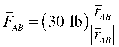Substitute the position vector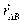and the magnitude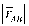and solve for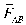.The force that cable AB exerts on the support B isand according to Newton’s third law, it is of equal magnitude and opposite direction to the force the cable exerts on A. Therefore,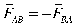.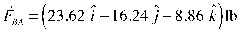For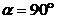:

Determine the coordinates of B, where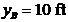.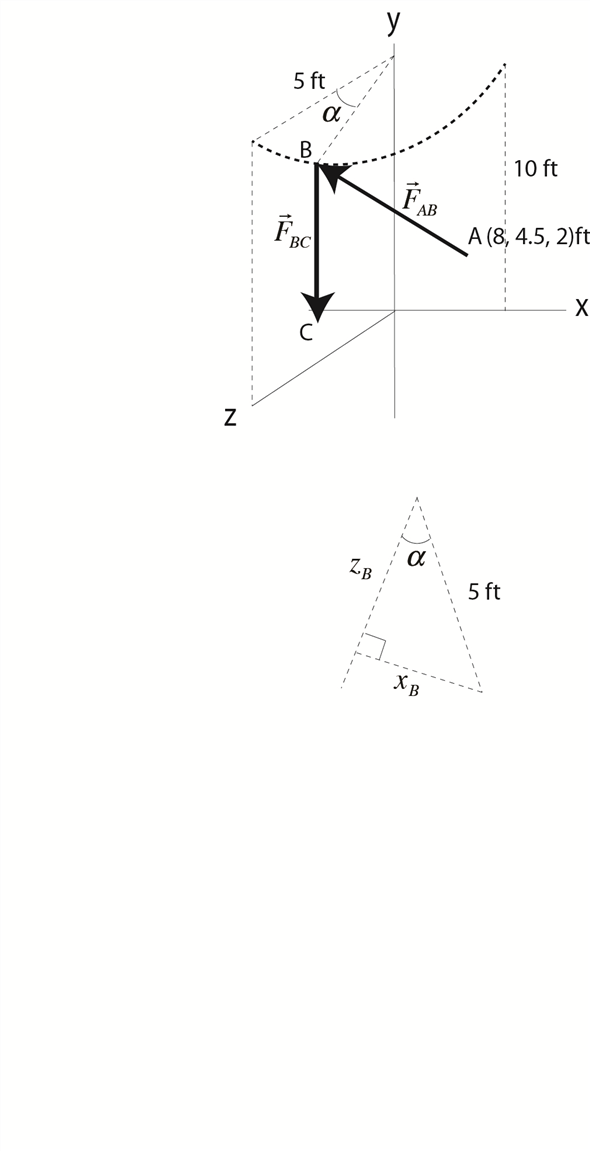Find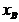using the bar radius (5 ft) and the angle,.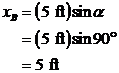Find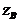using the bar radius (5 ft), the angle,.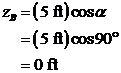Therefore, the coordinates of B are (5,10,0)ft.

Determine the position vector between A and B, using the relation,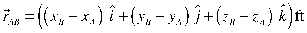Substitute the coordinates for point A, which are (8,4.5,2)ft, and the coordinates for point B, which are (5,10,0)ft.Useto calculate the magnitude of the vector,, using the relation,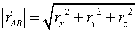Here,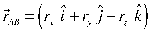.

Substitute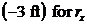,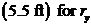, and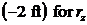.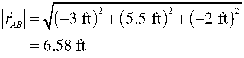Calculate the cable force exerted on A using the relation,Substitute the position vectorand the magnitudeand solve for.The force that cable AB exerts on the support B isand according to Newton’s third law, it is of equal magnitude and opposite direction to the force the cable exerts on A. Therefore,.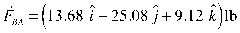Therefore,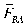, between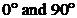, is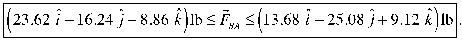The vertically hanging cable, BC, supports 1500 lbs.

Determine the force,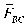.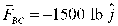Therefore, the force exerted by the cable BC on point B is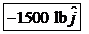, no matter what the angle of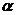.